x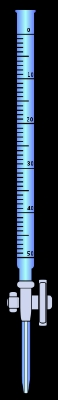Chemical equilibriumOverview

In a chemical reaction
Chemical reaction
A chemical reaction is a process that leads to the transformation of one set of chemical substances to another. Chemical reactions can be either spontaneous, requiring no input of energy, or non-spontaneous, typically following the input of some type of energy, such as heat, light or electricity...

, chemical equilibrium is the state in which the concentration
Concentration
In chemistry, concentration is defined as the abundance of a constituent divided by the total volume of a mixture. Four types can be distinguished: mass concentration, molar concentration, number concentration, and volume concentration...

s of the reactants and products have not yet changed with time. It occurs only in reversible reactions, and not in irreversible reactions. Usually, this state results when the forward reaction proceeds at the same rate as the reverse reaction
Reversible reaction
A reversible reaction is a chemical reaction that results in an equilibrium mixture of reactants and products. For a reaction involving two reactants and two products this can be expressed symbolically as...

. The reaction rate
Reaction rate
The reaction rate or speed of reaction for a reactant or product in a particular reaction is intuitively defined as how fast or slow a reaction takes place...

s of the forward and reverse reactions are generally not zero but, being equal, there are no net changes in the concentrations of the reactant and product.What the meaning of analytical eulibrium and how we can calculate itEncyclopedia
In a chemical reaction
Chemical reaction
A chemical reaction is a process that leads to the transformation of one set of chemical substances to another. Chemical reactions can be either spontaneous, requiring no input of energy, or non-spontaneous, typically following the input of some type of energy, such as heat, light or electricity...

, chemical equilibrium is the state in which the concentration
Concentration
In chemistry, concentration is defined as the abundance of a constituent divided by the total volume of a mixture. Four types can be distinguished: mass concentration, molar concentration, number concentration, and volume concentration...

s of the reactants and products have not yet changed with time. It occurs only in reversible reactions, and not in irreversible reactions. Usually, this state results when the forward reaction proceeds at the same rate as the reverse reaction
Reversible reaction
A reversible reaction is a chemical reaction that results in an equilibrium mixture of reactants and products. For a reaction involving two reactants and two products this can be expressed symbolically as...

. The reaction rate
Reaction rate
The reaction rate or speed of reaction for a reactant or product in a particular reaction is intuitively defined as how fast or slow a reaction takes place...

s of the forward and reverse reactions are generally not zero but, being equal, there are no net changes in the concentrations of the reactant and product. This process is called dynamic equilibrium
Dynamic equilibrium
A dynamic equilibrium exists once a reversible reaction ceases to change its ratio of reactants/products, but substances move between the chemicals at an equal rate, meaning there is no net change. It is a particular example of a system in a steady state...

.

## IntroductionThe concept of chemical equilibrium was developed after Berthollet (1803) found that some chemical reaction
Chemical reaction
A chemical reaction is a process that leads to the transformation of one set of chemical substances to another. Chemical reactions can be either spontaneous, requiring no input of energy, or non-spontaneous, typically following the input of some type of energy, such as heat, light or electricity...

s are reversible
Reversible reaction
A reversible reaction is a chemical reaction that results in an equilibrium mixture of reactants and products. For a reaction involving two reactants and two products this can be expressed symbolically as...

. For any reaction mixture to exist at equilibrium, the rates
Reaction rate
The reaction rate or speed of reaction for a reactant or product in a particular reaction is intuitively defined as how fast or slow a reaction takes place...

of the forward and backward (reverse) reactions are equal. In the following chemical equation
Chemical equation
A chemical equation is the symbolic representation of a chemical reaction where the reactant entities are given on the left hand side and the product entities on the right hand side. The coefficients next to the symbols and formulae of entities are the absolute values of the stoichiometric numbers...

with arrows pointing both ways to indicate equilibrium, A and B are reactant chemical species, S and T are product species, and α
Alpha (letter)
Alpha is the first letter of the Greek alphabet. In the system of Greek numerals it has a value of 1. It was derived from the Phoenician letter Aleph...

, β
Beta (letter)
Beta is the second letter of the Greek alphabet. In Ancient Greek, beta represented the voiced bilabial plosive . In Modern Greek, it represents the voiced labiodental fricative ....

, σ
Sigma
Sigma is the eighteenth letter of the Greek alphabet, and carries the 'S' sound. In the system of Greek numerals it has a value of 200. When used at the end of a word, and the word is not all upper case, the final form is used, e.g...

, and τ
Tau
Tau is the 19th letter of the Greek alphabet. In the system of Greek numerals it has a value of 300.The name in English is pronounced , but in modern Greek it is...

are the stoichiometric coefficients of the respective reactants and products: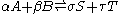The equilibrium position of a reaction is said to lie "far to the right" if, at equilibrium, nearly all the reactants are consumed. Conversely the equilibrium position is said to be "far to the left" if hardly any product is formed from the reactants.

Guldberg
Cato Maximilian Guldberg
Cato Maximilian Guldberg was a Norwegian mathematician and chemist.-Career:Guldberg worked at the Royal Frederick University. Together with his brother-in-law, Peter Waage, he proposed the law of mass action...

and Waage
Peter Waage
Peter Waage , the son of a ship's captain, was a significant Norwegian chemist and professor at the Royal Frederick University. Along with his brother-in-law Cato Maximilian Guldberg, he co-discovered and developed the law of mass action between 1864 and 1879.He grew up in Hidra...

(1865), building on Berthollet’s ideas, proposed the law of mass action: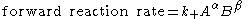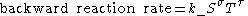where A, B, S and T are active masses
Activity (chemistry)
In chemical thermodynamics, activity is a measure of the “effective concentration” of a species in a mixture, meaning that the species' chemical potential depends on the activity of a real solution in the same way that it would depend on concentration for an ideal solution.By convention, activity...

and k+ and k are rate constants. Since at equilibrium forward and backward rates are equal: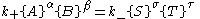and the ratio of the rate constants is also a constant, now known as an equilibrium constant.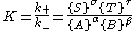By convention the products form the numerator.
However, the law of mass action is valid only for concerted one-step reactions that proceed through a single transition state
Transition state
The transition state of a chemical reaction is a particular configuration along the reaction coordinate. It is defined as the state corresponding to the highest energy along this reaction coordinate. At this point, assuming a perfectly irreversible reaction, colliding reactant molecules will always...

and is not valid in general because rate equations do not, in general, follow the stoichiometry
Stoichiometry
Stoichiometry is a branch of chemistry that deals with the relative quantities of reactants and products in chemical reactions. In a balanced chemical reaction, the relations among quantities of reactants and products typically form a ratio of whole numbers...

of the reaction as Guldberg and Waage had proposed (see, for example, nucleophilic aliphatic substitution by SN1 or reaction of hydrogen
Hydrogen
Hydrogen is the chemical element with atomic number 1. It is represented by the symbol H. With an average atomic weight of , hydrogen is the lightest and most abundant chemical element, constituting roughly 75% of the Universe's chemical elemental mass. Stars in the main sequence are mainly...

and bromine
Bromine
Bromine ") is a chemical element with the symbol Br, an atomic number of 35, and an atomic mass of 79.904. It is in the halogen element group. The element was isolated independently by two chemists, Carl Jacob Löwig and Antoine Jerome Balard, in 1825–1826...

to form hydrogen bromide
Hydrogen bromide
Hydrogen bromide is the diatomic molecule HBr. HBr is a gas at standard conditions. Hydrobromic acid forms upon dissolving HBr in water. Conversely, HBr can be liberated from hydrobromic acid solutions with the addition of a dehydration agent, but not by distillation. Hydrogen bromide and...

). Equality of forward and backward reaction rates, however, is a necessary condition
Necessary and sufficient conditions
In logic, the words necessity and sufficiency refer to the implicational relationships between statements. The assertion that one statement is a necessary and sufficient condition of another means that the former statement is true if and only if the latter is true.-Definitions:A necessary condition...

for chemical equilibrium, though it is not sufficient
Necessary and sufficient conditions
In logic, the words necessity and sufficiency refer to the implicational relationships between statements. The assertion that one statement is a necessary and sufficient condition of another means that the former statement is true if and only if the latter is true.-Definitions:A necessary condition...

to explain why equilibrium occurs.

Despite the failure of this derivation, the equilibrium constant for a reaction is indeed a constant, independent of the activities of the various species involved, though it does depend on temperature as observed by the van 't Hoff equation. Adding a catalyst will affect both the forward reaction and the reverse reaction in the same way and will not have an effect on the equilibrium constant. The catalyst will speed up both reactions thereby increasing the speed at which equilibrium is reached.

Although the macroscopic equilibrium concentrations are constant in time reactions do occur at the molecular level. For example, in the case of acetic acid
Acetic acid
Acetic acid is an organic compound with the chemical formula CH3CO2H . It is a colourless liquid that when undiluted is also called glacial acetic acid. Acetic acid is the main component of vinegar , and has a distinctive sour taste and pungent smell...

dissolved in water and forming acetate
Acetate
An acetate is a derivative of acetic acid. This term includes salts and esters, as well as the anion found in solution. Most of the approximately 5 billion kilograms of acetic acid produced annually in industry are used in the production of acetates, which usually take the form of polymers. In...

and hydronium
Hydronium
In chemistry, a hydronium ion is the cation , a type of oxonium ion produced by protonation of water. This cation is often used to represent the nature of the proton in aqueous solution, where the proton is highly solvated...

ions,
CH3CO2H + H2O CH3CO2 + H3O+

a proton may hop from one molecule of acetic acid on to a water molecule and then on to an acetate anion to form another molecule of acetic acid and leaving the number of acetic acid molecules unchanged. This is an example of dynamic equilibrium
Dynamic equilibrium
A dynamic equilibrium exists once a reversible reaction ceases to change its ratio of reactants/products, but substances move between the chemicals at an equal rate, meaning there is no net change. It is a particular example of a system in a steady state...

. Equilibria, like the rest of thermodynamics, are statistical phenomena, averages of microscopic behavior.

Le Chatelier's principle
Le Châtelier's principle
In chemistry, Le Chatelier's principle, also called the Chatelier's principle, can be used to predict the effect of a change in conditions on a chemical equilibrium. The principle is named after Henry Louis Le Chatelier and sometimes Karl Ferdinand Braun who discovered it independently...

(1884) gives an idea of the behavior of an equilibrium system when changes to its reaction conditions occur. If a dynamic equilibrium is disturbed by changing the conditions, the position of equilibrium moves to partially reverse the change. For example, adding more S from the outside will cause an excess of products, and the system will try to counteract this by increasing the reverse reaction and pushing the equilibrium point backward (though the equilibrium constant will stay the same).

If mineral acid
Mineral acid
A mineral acid is an acid derived from one or more inorganic compounds. A mineral acid is not organic and all mineral acids release hydrogen ions when dissolved in water.-Characteristics:...

is added to the acetic acid mixture, increasing the concentration of hydronium ion, the amount of dissociation must decrease as the reaction is driven to the left in accordance with this principle. This can also be deduced from the equilibrium constant expression for the reaction: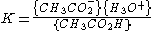If {H3O+} increases {CH3CO2H} must increase and {CH3CO2} must decrease. The H2O is left out as it is a pure liquid and its concentration is undefined.

A quantitative version is given by the reaction quotient
Reaction quotient
In chemistry, a reaction quotient: Qr is a function of the activities or concentrations of the chemical species involved in a chemical reaction. In the special case that the reaction is at equilibrium the reaction quotient is equal to the equilibrium constant....

.

J. W. Gibbs
Josiah Willard Gibbs
Josiah Willard Gibbs was an American theoretical physicist, chemist, and mathematician. He devised much of the theoretical foundation for chemical thermodynamics as well as physical chemistry. As a mathematician, he invented vector analysis . Yale University awarded Gibbs the first American Ph.D...

suggested in 1873 that equilibrium is attained when the Gibbs energy
Chemical potential
Chemical potential, symbolized by μ, is a measure first described by the American engineer, chemist and mathematical physicist Josiah Willard Gibbs. It is the potential that a substance has to produce in order to alter a system...

of the system is at its minimum value (assuming the reaction is carried out under constant pressure). What this means is that the derivative of the Gibbs energy with respect to reaction coordinate
Reaction coordinate
In chemistry, a reaction coordinate is an abstract one-dimensional coordinate which represents progress along a reaction pathway. It is usually a geometric parameter that changes during the conversion of one or more molecular entities....

(a measure of the extent of reaction that has occurred, ranging from zero for all reactants to a maximum for all products) vanishes, signalling a stationary point
Stationary point
In mathematics, particularly in calculus, a stationary point is an input to a function where the derivative is zero : where the function "stops" increasing or decreasing ....

. This derivative is usually called, for certain technical reasons, the Gibbs energy change. This criterion is both necessary and sufficient. If a mixture is not at equilibrium, the liberation of the excess Gibbs energy (or Helmholtz energy at constant volume reactions) is the “driving force” for the composition of the mixture to change until equilibrium is reached. The equilibrium constant can be related to the standard Gibbs energy change for the reaction by the equation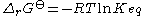where R is the universal gas constant and T the temperature
Temperature
Temperature is a physical property of matter that quantitatively expresses the common notions of hot and cold. Objects of low temperature are cold, while various degrees of higher temperatures are referred to as warm or hot...

.

When the reactants are dissolved
Solution
In chemistry, a solution is a homogeneous mixture composed of only one phase. In such a mixture, a solute is dissolved in another substance, known as a solvent. The solvent does the dissolving.- Types of solutions :...

in a medium of high ionic strength
Ionic strength
The ionic strength of a solution is a measure of the concentration of ions in that solution. Ionic compounds, when dissolved in water, dissociate into ions. The total electrolyte concentration in solution will affect important properties such as the dissociation or the solubility of different salts...

the quotient of activity coefficient
Activity coefficient
An activity coefficient is a factor used in thermodynamics to account for deviations from ideal behaviour in a mixture of chemical substances. In an ideal mixture, the interactions between each pair of chemical species are the same and, as a result, properties of the mixtures can be expressed...

s may be taken to be constant. In that case the concentration quotient, Kc,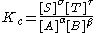where [A] is the concentration
Concentration
In chemistry, concentration is defined as the abundance of a constituent divided by the total volume of a mixture. Four types can be distinguished: mass concentration, molar concentration, number concentration, and volume concentration...

of A, etc., is independent of the analytical concentration of the reactants. For this reason, equilibrium constants for solution
Solution
In chemistry, a solution is a homogeneous mixture composed of only one phase. In such a mixture, a solute is dissolved in another substance, known as a solvent. The solvent does the dissolving.- Types of solutions :...

s are usually determined
Determination of equilibrium constants
Equilibrium constants are determined in order to quantify chemical equilibria. When an equilibrium constant is expressed as a concentration quotient,K=\frac...

in media of high ionic strength. Kc varies with ionic strength, temperature and pressure (or volume). Likewise Kp for gases depends on partial pressure
Partial pressure
In a mixture of ideal gases, each gas has a partial pressure which is the pressure which the gas would have if it alone occupied the volume. The total pressure of a gas mixture is the sum of the partial pressures of each individual gas in the mixture....

. These constants are easier to measure and encountered in high-school chemistry courses.

## Thermodynamics

The relation between the Gibbs energy and the equilibrium constant can be found by considering chemical potentials.
At constant temperature and pressure the function G Gibbs free energy
Gibbs free energy
In thermodynamics, the Gibbs free energy is a thermodynamic potential that measures the "useful" or process-initiating work obtainable from a thermodynamic system at a constant temperature and pressure...

for the reaction, depends only with the extent of reaction: ξ and can only decrease according to the second law of thermodynamics
Second law of thermodynamics
The second law of thermodynamics is an expression of the tendency that over time, differences in temperature, pressure, and chemical potential equilibrate in an isolated physical system. From the state of thermodynamic equilibrium, the law deduced the principle of the increase of entropy and...

. It means that the derivative of G with ξ must be negative if the reaction happens; at the equilibrium the derivative being equal to zero.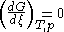: equilibrium

At constant volume, one must consider the Helmholtz free energy
Helmholtz free energy
In thermodynamics, the Helmholtz free energy is a thermodynamic potential that measures the “useful” work obtainable from a closed thermodynamic system at a constant temperature and volume...

for the reaction: A.

In this article only the constant pressure case is considered. The constant volume case is important in geochemistry
Geochemistry
The field of geochemistry involves study of the chemical composition of the Earth and other planets, chemical processes and reactions that govern the composition of rocks, water, and soils, and the cycles of matter and energy that transport the Earth's chemical components in time and space, and...

and atmospheric chemistry
Atmospheric chemistry
Atmospheric chemistry is a branch of atmospheric science in which the chemistry of the Earth's atmosphere and that of other planets is studied. It is a multidisciplinary field of research and draws on environmental chemistry, physics, meteorology, computer modeling, oceanography, geology and...

where pressure variations are significant. Note that, if reactants and products were in standard state (completely pure), then there would be no reversibility and no equilibrium. The mixing of the products and reactants contributes a large entropy (known as entropy of mixing
Entropy of mixing
In thermodynamics the entropy of mixing is the increase in the total entropy of a compound system, when different and chemically non-reacting chemical substances or material components are mixed by removing partition between the system's initially separate volumes...

) to states containing equal mixture of products and reactants. The combination of the standard Gibbs energy change and the Gibbs energy of mixing determines the equilibrium state.

In general an equilibrium system is defined by writing an equilibrium equation for the reactionIn order to meet the thermodynamic condition for equilibrium, the Gibbs energy must be stationary, meaning that the derivative of G with respect to the extent of reaction: ξ, must be zero. It can be shown that in this case, the sum of chemical potential
Chemical potential
Chemical potential, symbolized by μ, is a measure first described by the American engineer, chemist and mathematical physicist Josiah Willard Gibbs. It is the potential that a substance has to produce in order to alter a system...

s of the products is equal to the sum of those corresponding to the reactants. Therefore, the sum of the Gibbs energies of the reactants must be the equal to the sum of the Gibbs energies of the products.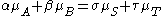where μ
Mu (letter)
Carlos Alberto Vives Restrepo is a Grammy Award and three-time Latin Grammy Award winning-Colombian singer, composer and actor.-Biography:...

is in this case a partial molar Gibbs energy, a chemical potential
Chemical potential
Chemical potential, symbolized by μ, is a measure first described by the American engineer, chemist and mathematical physicist Josiah Willard Gibbs. It is the potential that a substance has to produce in order to alter a system...

. The chemical potential of a reagent A is a function of the
activity
Activity (chemistry)
In chemical thermodynamics, activity is a measure of the “effective concentration” of a species in a mixture, meaning that the species' chemical potential depends on the activity of a real solution in the same way that it would depend on concentration for an ideal solution.By convention, activity...

, {A} of that reagent.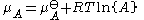, (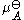is the standard chemical potential ).

Substituting expressions like this into the Gibbs energy equation: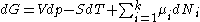in the case of a closed system
Closed system
-In physics:In thermodynamics, a closed system can exchange energy , but not matter, with its surroundings.In contrast, an isolated system cannot exchange any of heat, work, or matter with the surroundings, while an open system can exchange all of heat, work and matter.For a simple system, with...

.
Now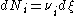(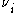corresponds to the Stoichiometric coefficient and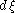is the differential
Differential of a function
In calculus, the differential represents the principal part of the change in a function y = ƒ with respect to changes in the independent variable. The differential dy is defined bydy = f'\,dx,...

of the extent of reaction ).

At constant pressure and temperature we obtain: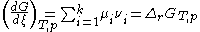which corresponds to the Gibbs free energy change for the reaction .

This results in: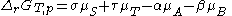.

By substituting the chemical potentials: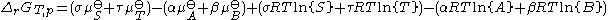,

the relationship becomes: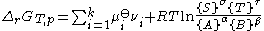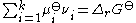: which is the standard Gibbs energy change for the reaction. It is a constant at a given temperature, which can be calculated, using thermodynamical tables.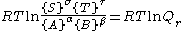(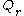is the reaction quotient
Reaction quotient
In chemistry, a reaction quotient: Qr is a function of the activities or concentrations of the chemical species involved in a chemical reaction. In the special case that the reaction is at equilibrium the reaction quotient is equal to the equilibrium constant....

when the system is not at equilibrium ).

Therefore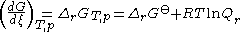At equilibrium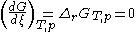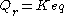; the reaction quotient becomes equal to the equilibrium constant.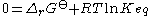andObtaining the value of the standard Gibbs energy change, allows the calculation of the equilibrium constant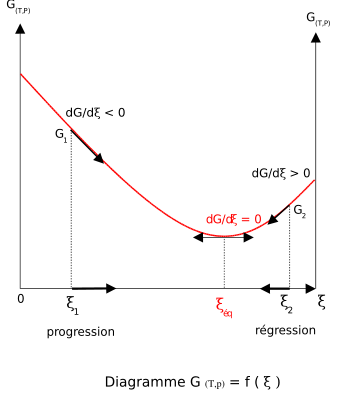### Addition of reactants or products

For a reactional system at equilibrium: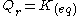;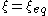.
If are modified activities of constituents, the value of the reaction quotient changes and becomes different from the equilibrium constant: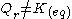and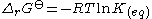then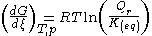• If activity of a reagent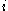increases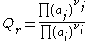, the reaction quotient decreases.
then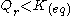and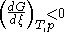: The reaction will shift to the right (i.e. in the forward direction, and thus more products will form).
• If activity of a product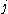increases
then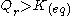and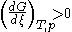: The reaction will shift to the left (i.e. in the reverse direction, and thus less products will form).

Note that activities and equilibrium constants are dimensionless numbers.

### Treatment of activity

The expression for the equilibrium constant can be rewritten as the product of a concentration quotient, Kc and an activity coefficient
Activity coefficient
An activity coefficient is a factor used in thermodynamics to account for deviations from ideal behaviour in a mixture of chemical substances. In an ideal mixture, the interactions between each pair of chemical species are the same and, as a result, properties of the mixtures can be expressed...

quotient, Γ.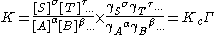[A] is the concentration of reagent A, etc. It is possible in principle to obtain values of the activity coefficients, γ. For solutions, equations such as the Debye-Hückel equation
Debye-Hückel equation
The Debye–Hückel equation and Debye–Hückel limiting law, were derived by Peter Debye and Erich Hückel, who developed a theory with which to calculate activity coefficients of electrolyte solutions. Activities, rather than concentrations, are needed in many chemical calculations because solutions...

or extensions such as Davies equation
Davies equation
The Davies equation is an empirical extension of Debye–Hückel theory which can be used to calculate activity coefficients of electrolyte solutions at relatively high concentrations. The equation, originally published in 1938, was refined by fitting to experimental data...

Specific ion interaction theory
Specific ion interaction theory
Specific ion Interaction Theory is a theory used to estimate single-ion activity coefficients in electrolyte solutions at relatively high concentrations. It does so by taking into consideration interaction coefficients between the various ions present in solution...

or Pitzer equations
Pitzer equations
Pitzer equations are important for the understanding of the behaviour of ions dissolved in natural waters such as rivers, lakes and sea-water. The parameters of the Pitzer equations are linear combinations of parameters, of a virial expansion of the excess Gibbs free energy, which characterise...

may be used.Software (below). However this is not always possible. It is common practice to assume that Γ is a constant, and to use the concentration quotient in place of the thermodynamic equilibrium constant. It is also general practice to use the term equilibrium constant instead of the more accurate concentration quotient. This practice will be followed here.

For reactions in the gas phase partial pressure
Partial pressure
In a mixture of ideal gases, each gas has a partial pressure which is the pressure which the gas would have if it alone occupied the volume. The total pressure of a gas mixture is the sum of the partial pressures of each individual gas in the mixture....

is used in place of concentration and fugacity coefficient in place of activity coefficient. In the real world, for example, when making ammonia
Haber process
The Haber process, also called the Haber–Bosch process, is the nitrogen fixation reaction of nitrogen gas and hydrogen gas, over an enriched iron or ruthenium catalyst, which is used to industrially produce ammonia....

in industry, fugacity coefficients must be taken into account. Fugacity, f, is the product of partial pressure and fugacity coefficient. The chemical potential of a species in the gas phase is given by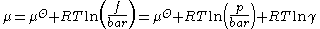so the general expression defining an equilibrium constant is valid for both solution and gas phases.

### Concentration quotients

In aqueous solution, equilibrium constants are usually determined in the presence of an "inert" electrolyte such as sodium nitrate
Sodium nitrate
Sodium nitrate is the chemical compound with the formula NaNO3. This salt, also known as Chile saltpeter or Peru saltpeter to distinguish it from ordinary saltpeter, potassium nitrate, is a white solid which is very soluble in water...

NaNO3 or Potassium perchlorate
Potassium perchlorate
Potassium perchlorate is the inorganic salt with the chemical formula KClO4. Like other perchlorates, this salt is a strong oxidizer and potentially reacts with many organic substances...

KClO4. The ionic strength
Ionic strength
The ionic strength of a solution is a measure of the concentration of ions in that solution. Ionic compounds, when dissolved in water, dissociate into ions. The total electrolyte concentration in solution will affect important properties such as the dissociation or the solubility of different salts...

, I, of a solution containing a dissolved salt, X+Y-, is given by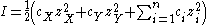where c stands for concentration, z stands for ionic charge and the sum is taken over all the species in equilibrium. When the concentration of dissolved salt is much higher than the analytical concentrations of the reagents, the ionic strength is effectively constant. Since activity coefficients depend on ionic strength the activity coefficients of the species are effectively independent of concentration. Thus, the assumption that Γ
Gamma
Gamma is the third letter of the Greek alphabet. In the system of Greek numerals it has a value of 3. It was derived from the Phoenician letter Gimel . Letters that arose from Gamma include the Roman C and G and the Cyrillic letters Ge Г and Ghe Ґ.-Greek:In Ancient Greek, gamma represented a...

is constant is justified. The concentration quotient is a simple multiple of the equilibrium constant.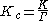However, Kc will vary with ionic strength. If it is measured at a series of different ionic strengths the value can be extrapolated to zero ionic strength. The concentration quotient obtained in this manner is known, paradoxically, as a thermodynamic equilibrium constant.

To use a published value of an equilibrium constant in conditions of ionic strength different from the conditions used in its determination, the value should be adjustedSoftware (below).

### Metastable mixtures

A mixture may be appear to have no tendency to change, though it is not at equilibrium. For example, a mixture of SO2
Sulfur dioxide
Sulfur dioxide is the chemical compound with the formula . It is released by volcanoes and in various industrial processes. Since coal and petroleum often contain sulfur compounds, their combustion generates sulfur dioxide unless the sulfur compounds are removed before burning the fuel...

and O2
Oxygen
Oxygen is the element with atomic number 8 and represented by the symbol O. Its name derives from the Greek roots ὀξύς and -γενής , because at the time of naming, it was mistakenly thought that all acids required oxygen in their composition...

is metastable as there is a kinetic barrier
Activation energy
In chemistry, activation energy is a term introduced in 1889 by the Swedish scientist Svante Arrhenius that is defined as the energy that must be overcome in order for a chemical reaction to occur. Activation energy may also be defined as the minimum energy required to start a chemical reaction...

to formation of the product, SO3
Sulfur trioxide
Sulfur trioxide is the chemical compound with the formula SO3. In the gaseous form, this species is a significant pollutant, being the primary agent in acid rain. It is prepared on massive scales as a precursor to sulfuric acid.-Structure and bonding:Gaseous SO3 is a trigonal planar molecule of...

.
2SO2 + O2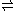2SO3

The barrier can be overcome when a catalyst
Catalysis
Catalysis is the change in rate of a chemical reaction due to the participation of a substance called a catalyst. Unlike other reagents that participate in the chemical reaction, a catalyst is not consumed by the reaction itself. A catalyst may participate in multiple chemical transformations....

is also present in the mixture as in the contact process
Contact process
The contact process is the current method of producing sulphuric acid in the high concentrations needed for industrial processes. Platinum was formerly employed as a catalyst for the reaction, but as it is susceptible to poisoning by arsenic impurities in the sulfur feedstock, vanadium oxide is...

, but the catalyst does not affect the equilibrium concentrations.

Likewise, the formation of bicarbonate
Bicarbonate
In inorganic chemistry, bicarbonate is an intermediate form in the deprotonation of carbonic acid...

from carbon dioxide
Carbon dioxide
Carbon dioxide is a naturally occurring chemical compound composed of two oxygen atoms covalently bonded to a single carbon atom...

and water
Water
Water is a chemical substance with the chemical formula H2O. A water molecule contains one oxygen and two hydrogen atoms connected by covalent bonds. Water is a liquid at ambient conditions, but it often co-exists on Earth with its solid state, ice, and gaseous state . Water also exists in a...

is very slow under normal conditions
CO2 + 2H2OHCO3- +H3O+

but almost instantaneous in the presence of the catalytic enzyme
Enzyme
Enzymes are proteins that catalyze chemical reactions. In enzymatic reactions, the molecules at the beginning of the process, called substrates, are converted into different molecules, called products. Almost all chemical reactions in a biological cell need enzymes in order to occur at rates...

carbonic anhydrase
Carbonic anhydrase
The carbonic anhydrases form a family of enzymes that catalyze the rapid interconversion of carbon dioxide and water to bicarbonate and protons , a reversible reaction that occurs rather slowly in the absence of a catalyst...

.

## Pure compounds

When pure substances (liquids or solids) are involved in equilibria they do not appear in the equilibrium equation

Applying the general formula for an equilibrium constant to the specific case of acetic acid one obtains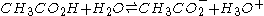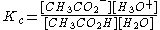It may be assumed that the concentration of water is constant. This assumption will be valid for all but very concentrated solutions. The equilibrium constant expression is therefore usually written as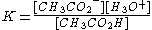where now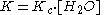a constant factor is incorporated into the equilibrium constant.

A particular case is the self-ionization of water
Self-ionization of water
The self-ionization of water is the chemical reaction in which a proton is transferred from one water molecule to another, in pure water or an aqueous solution, to create the two ions, hydronium, H3O+ and hydroxide, OH−...

itself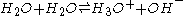The self-ionization constant of water is defined as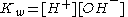It is perfectly legitimate to write [H+] for the hydronium ion concentration, since the state of solvation
Solvation
Solvation, also sometimes called dissolution, is the process of attraction and association of molecules of a solvent with molecules or ions of a solute...

of the proton is constant (in dilute solutions) and so does not affect the equilibrium concentrations. Kw varies with variation in ionic strength and/or temperature.

The concentrations of H+ and OH- are not independent quantities. Most commonly [OH-] is replaced by Kw[H+]−1 in equilibrium constant expressions which would otherwise hydroxide
Hydroxide
Hydroxide is a diatomic anion with chemical formula OH−. It consists of an oxygen and a hydrogen atom held together by a covalent bond, and carrying a negative electric charge. It is an important but usually minor constituent of water. It functions as a base, as a ligand, a nucleophile, and a...

.

Solids also do not appear in the equilibrium equation. An example is the Boudouard reaction
Boudouard reaction
The Boudouard reaction is the redox reaction of a chemical equilibrium mixture of carbon monoxide and carbon dioxide at a given temperature. It is the disproportionation of carbon monoxide into carbon dioxide and graphite or its reverse:...

: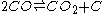for which the equation (without solid carbon) is written as: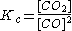## Multiple equilibria

Consider the case of a dibasic acid H2A. When dissolved in water, the mixture will contain H2A, HA- and A2-. This equilibrium can be split into two steps in each of which one proton is liberated.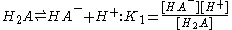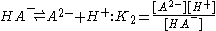K1 and K2 are examples of stepwise equilibrium constants. The overall equilibrium constant,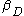, is product of the stepwise constants.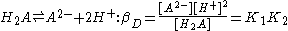Note that these constants are dissociation constants
Acid dissociation constant
An acid dissociation constant, Ka, is a quantitative measure of the strength of an acid in solution. It is the equilibrium constant for a chemical reaction known as dissociation in the context of acid-base reactions...

because the products on the right hand side of the equilibrium expression are dissociation products. In many systems, it is preferable to use association constants.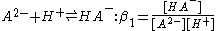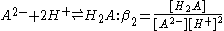β1 and β2 are examples of association constants. Clearly β1 = 1/K2 and β2 = 1/βD; lg β1 = pK2 and lg β2 = pK2 + pK1
For multiple equilibrium systems, also see: theory of Response reactions
Response reactions
The theory of response reactions or response equilibria was elaborated for the thermodynamic systems in which more than one equilibrium is established simultaneously. It is based on detailed analysis of the Hessian determinant. The theory derives the sensitivity coefficient as the sum of the...

.

## Effect of temperature

The effect of changing temperature on an equilibrium constant is given by the van 't Hoff equation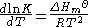Thus, for exothermic
Exothermic
In thermodynamics, the term exothermic describes a process or reaction that releases energy from the system, usually in the form of heat, but also in the form of light , electricity , or sound...

reactions, (ΔH is negative) K decreases with an increase in temperature, but, for endothermic
Endothermic
In thermodynamics, the word endothermic describes a process or reaction in which the system absorbs energy from the surroundings in the form of heat. Its etymology stems from the prefix endo- and the Greek word thermasi,...

reactions, (ΔH is positive) K increases with an increase temperature. An alternative formulation is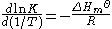At first sight this appears to offer a means of obtaining the standard molar enthalpy of the reaction by studying the variation of K with temperature. In practice, however, the method is unreliable because error propagation almost always gives very large errors on the values calculated in this way.

## Types of equilibrium

1. In the gas phase. Rocket engine
Rocket engine
A rocket engine, or simply "rocket", is a jet engineRocket Propulsion Elements; 7th edition- chapter 1 that uses only propellant mass for forming its high speed propulsive jet. Rocket engines are reaction engines and obtain thrust in accordance with Newton's third law...

s
2. The industrial synthesis such as ammonia
Ammonia
Ammonia is a compound of nitrogen and hydrogen with the formula . It is a colourless gas with a characteristic pungent odour. Ammonia contributes significantly to the nutritional needs of terrestrial organisms by serving as a precursor to food and fertilizers. Ammonia, either directly or...

in the Haber-Bosch process (depicted right) takes place through a succession of equilibrium steps including adsorption
Adsorption is the adhesion of atoms, ions, biomolecules or molecules of gas, liquid, or dissolved solids to a surface. This process creates a film of the adsorbate on the surface of the adsorbent. It differs from absorption, in which a fluid permeates or is dissolved by a liquid or solid...

processes.3. atmospheric chemistry
Atmospheric chemistry
Atmospheric chemistry is a branch of atmospheric science in which the chemistry of the Earth's atmosphere and that of other planets is studied. It is a multidisciplinary field of research and draws on environmental chemistry, physics, meteorology, computer modeling, oceanography, geology and...

4. Seawater and other natural waters: Chemical oceanography
Chemical oceanography
Chemical oceanography is the study of ocean chemistry: the behavior of the chemical elements within the Earth's oceans. The ocean is unique in that it contains - in greater or lesser quantities - nearly every element in the periodic table....

5. Distribution between two phases
1. LogD-Distribution coefficient: Important for pharmaceuticals where lipophilicity is a significant property of a drug
2. Liquid-liquid extraction
Liquid-liquid extraction
Liquid–liquid extraction, also known as solvent extraction and partitioning, is a method to separate compounds based on their relative solubilities in two different immiscible liquids, usually water and an organic solvent. It is an extraction of a substance from one liquid phase into another liquid...

, Ion exchange
Ion exchange
Ion exchange is an exchange of ions between two electrolytes or between an electrolyte solution and a complex. In most cases the term is used to denote the processes of purification, separation, and decontamination of aqueous and other ion-containing solutions with solid polymeric or mineralic 'ion...

, Chromatography
Chromatography
Chromatography is the collective term for a set of laboratory techniques for the separation of mixtures....

3. Solubility product
Solubility equilibrium
Solubility equilibrium is a type of dynamic equilibrium. It exists when a chemical compound in the solid state is in chemical equilibrium with a solution of that compound. The solid may dissolve unchanged, with dissociation or with chemical reaction with another constituent of the solvent, such as...

4. Uptake and release of oxygen by haemoglobin in blood
6. Acid/base equilibria: Acid dissociation constant
Acid dissociation constant
An acid dissociation constant, Ka, is a quantitative measure of the strength of an acid in solution. It is the equilibrium constant for a chemical reaction known as dissociation in the context of acid-base reactions...

, hydrolysis
Hydrolysis
Hydrolysis is a chemical reaction during which molecules of water are split into hydrogen cations and hydroxide anions in the process of a chemical mechanism. It is the type of reaction that is used to break down certain polymers, especially those made by condensation polymerization...

, buffer solution
Buffer solution
A buffer solution is an aqueous solution consisting of a mixture of a weak acid and its conjugate base or a weak base and its conjugate acid. It has the property that the pH of the solution changes very little when a small amount of strong acid or base is added to it. Buffer solutions are used as a...

s, indicators
PH indicator
A pH indicator is a halochromic chemical compound that is added in small amounts to a solution so that the pH of the solution can be determined visually. Hence a pH indicator is a chemical detector for hydronium ions or hydrogen ions in the Arrhenius model. Normally, the indicator causes the...

, acid-base homeostasis
Acid-base homeostasis
Acid–base homeostasis is the part of human homeostasis concerning the proper balance between acids and bases, in other words, the pH. The body is very sensitive to its pH level, so strong mechanisms exist to maintain it...

7. Metal-ligand complexation: sequestering agents
Chelation
Chelation is the formation or presence of two or more separate coordinate bonds between apolydentate ligand and a single central atom....

, chelation therapy
Chelation therapy
Chelation therapy is the administration of chelating agents to remove heavy metals from the body. For the most common forms of heavy metal intoxication—those involving lead, arsenic or mercury—the standard of care in the United States dictates the use of dimercaptosuccinic acid...

, MRI contrast reagents
Magnetic resonance imaging
Magnetic resonance imaging , nuclear magnetic resonance imaging , or magnetic resonance tomography is a medical imaging technique used in radiology to visualize detailed internal structures...

, Schlenk equilibrium
Schlenk equilibrium
The Schlenk equilibrium is a chemical equilibrium named after its discoverer Wilhelm Schlenk taking place in solutions of Grignard reagents.The process described is an equilibrium between two equivalents of an alkyl or aryl magnesium halide on the left of the equation and on the right side, one...

Host-guest chemistry
In supramolecular chemistry, host-guest chemistry describes complexes that are composed of two or more molecules or ions that are held together in unique structural relationships by forces other than those of full covalent bonds. Host-guest chemistry encompasses the idea of molecular recognition...

, supramolecular chemistry
Supramolecular chemistry
Supramolecular chemistry refers to the area of chemistry beyond the molecules and focuses on the chemical systems made up of a discrete number of assembled molecular subunits or components...

, molecular recognition
Molecular recognition
The term molecular recognition refers to the specific interaction between two or more molecules through noncovalent bonding such as hydrogen bonding, metal coordination, hydrophobic forces, van der Waals forces, π-π interactions, electrostatic and/or electromagnetic effects...

, dinitrogen tetroxide
Dinitrogen tetroxide
Dinitrogen tetroxide is the chemical compound N2O4. It is a useful reagent in chemical synthesis. It forms an equilibrium mixture with nitrogen dioxide; some call this mixture dinitrogen tetroxide, while some call it nitrogen dioxide.Dinitrogen tetroxide is a powerful oxidizer, making it highly...

9. In certain oscillating reactions, the approach to equilibrium is not asymptotically but in the form of a damped oscillation .
10. The related Nernst equation
Nernst equation
In electrochemistry, the Nernst equation is an equation that can be used to determine the equilibrium reduction potential of a half-cell in an electrochemical cell. It can also be used to determine the total voltage for a full electrochemical cell...

in electrochemistry gives the difference in electrode potential as a function of redox concentrations.
11. When molecules on each side of the equilibrium are able to further react irreversibly in secondary reactions, the final product ratio is determined according to the Curtin-Hammett principle
Curtin-Hammett principle
The Curtin–Hammett principle is a principle in chemical kinetics proposed by David Yarrow Curtin and Louis Plack Hammett. It states that, for a reaction that has a pair of reactive intermediates or reactants that interconvert rapidly , each going irreversibly to a different product, the product...

.

In these applications, terms such as stability constant, formation constant, binding constant, affinity constant, association/dissociation constant are used. In biochemistry, it is common to give units for binding constants, which serve to define the concentration units used when the constant’s value was determined.

## Composition of a mixture

When the only equilibrium is that of the formation of a 1:1 adduct as the composition of a mixture, there are any number of ways that the composition of a mixture can be calculated. For example, see ICE table
ICE table
An ICE table, ICE chart, or ICE box is a tabular system of keeping track of changing concentrations in an equilibrium reaction. ICE stands for "Initial, Change, Equilibrium". It is used in chemistry to keep track of the changes in amount of substance of the reactants and also organize a set of...

for a traditional method of calculating the pH of a solution of a weak acid.

There are three approaches to the general calculation of the composition of a mixture at equilibrium.
1. The most basic approach is to manipulate the various equilibrium constants until the desired concentrations are expressed in terms of measured equilibrium constants (equivalent to measuring chemical potentials) and initial conditions.
2. Minimize the Gibbs energy of the system.
3. Satisfy the equation of mass balance
Mass balance
A mass balance is an application of conservation of mass to the analysis of physical systems. By accounting for material entering and leaving a system, mass flows can be identified which might have been unknown, or difficult to measure without this technique...

. The equations of mass balance are simply statements that demonstrate that the total concentration of each reactant must be constant by the law of conservation of mass
Conservation of mass
The law of conservation of mass, also known as the principle of mass/matter conservation, states that the mass of an isolated system will remain constant over time...

.

### Mass-balance equations

In general, the calculations are rather complicated. For instance, in the case of a dibasic acid, H2A dissolved in water the two reactants can be specified as the conjugate base, A2-, and the proton
Hydronium
In chemistry, a hydronium ion is the cation , a type of oxonium ion produced by protonation of water. This cation is often used to represent the nature of the proton in aqueous solution, where the proton is highly solvated...

, H+. The following equations of mass-balance could apply equally well to a base such as 1,2-diaminoethane, in which case the base itself is designated as the reactant A: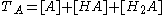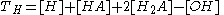With TA the total concentration of species A. Note that it is customary to omit the ionic charges when writing and using these equations.

When the equilibrium constants are known and the total concentrations are specified there are two equations in two unknown "free concentrations" [A] and [H]. This follows from the fact that [HA]= β1[A][H], [H2A]= β2[A][H]2 and [OH] = Kw[H]−1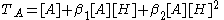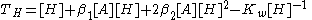so the concentrations of the "complexes" are calculated from the free concentrations and the equilibrium constants.
General expressions applicable to all systems with two reagents, A and B would be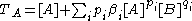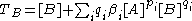It is easy to see how this can be extended to three or more reagents.

#### Polybasic acids

The composition of solutions containing reactants A and H is easy to calculate as a function of p[H
PH
In chemistry, pH is a measure of the acidity or basicity of an aqueous solution. Pure water is said to be neutral, with a pH close to 7.0 at . Solutions with a pH less than 7 are said to be acidic and solutions with a pH greater than 7 are basic or alkaline...

]. When [H] is known, the free concentration [A] is calculated from the mass-balance equation in A. Here is an example of the results that can be obtained.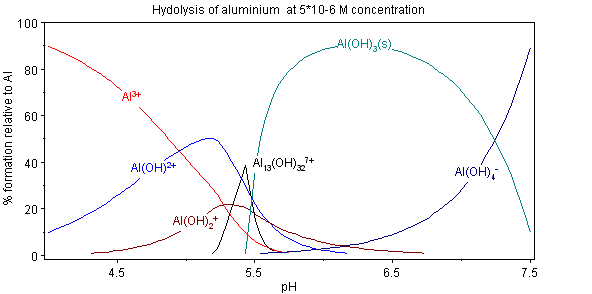This diagram, for the hydrolysis of the aluminium
Aluminium
Aluminium or aluminum is a silvery white member of the boron group of chemical elements. It has the symbol Al, and its atomic number is 13. It is not soluble in water under normal circumstances....

Lewis acid
Lewis acid
]The term Lewis acid refers to a definition of acid published by Gilbert N. Lewis in 1923, specifically: An acid substance is one which can employ a lone pair from another molecule in completing the stable group of one of its own atoms. Thus, H+ is a Lewis acid, since it can accept a lone pair,...

Al3+aq shows the species concentrations for a 5×10−6M solution of an aluminium salt as a function of pH. Each concentration is shown as a percentage of the total aluminium.

#### Solution and precipitation

The diagram above illustrates the point that a precipitate
Precipitation (chemistry)
Precipitation is the formation of a solid in a solution or inside anothersolid during a chemical reaction or by diffusion in a solid. When the reaction occurs in a liquid, the solid formed is called the precipitate, or when compacted by a centrifuge, a pellet. The liquid remaining above the solid...

that is not one of the main species in the solution equilibrium may be formed. At pH just below 5.5 the main species present in a 5μM solution of Al3+ are aluminium hydroxide
Aluminium hydroxide
Aluminium hydroxide, Al3, ATH, sometimes erroneously called Hydrate of alumina, is found in nature as the mineral gibbsite and its three, much more rare forms, polymorphs: bayerite, doyleite and nordstrandite. Closely related are aluminium oxide hydroxide, AlO, and aluminium oxide, Al2O3,...

s Al(OH)2+, Al(OH)2+ and Al13(OH)327+, but on raising the pH Al(OH)3
Aluminium hydroxide
Aluminium hydroxide, Al3, ATH, sometimes erroneously called Hydrate of alumina, is found in nature as the mineral gibbsite and its three, much more rare forms, polymorphs: bayerite, doyleite and nordstrandite. Closely related are aluminium oxide hydroxide, AlO, and aluminium oxide, Al2O3,...

precipitates from the solution. This occurs because Al(OH)3 has a very large lattice energy
Lattice energy
The lattice energy of an ionic solid is a measure of the strength of bonds in that ionic compound. It is usually defined as the enthalpy of formation of the ionic compound from gaseous ions and as such is invariably exothermic. Lattice energy may also be defined as the energy required to completely...

. As the pH rises more and more Al(OH)3 comes out of solution. This is an example of Le Chatelier's principle
Le Châtelier's principle
In chemistry, Le Chatelier's principle, also called the Chatelier's principle, can be used to predict the effect of a change in conditions on a chemical equilibrium. The principle is named after Henry Louis Le Chatelier and sometimes Karl Ferdinand Braun who discovered it independently...

in action: Increasing the concentration of the hydroxide ion causes more aluminium hydroxide to precipitate, which removes hydroxide from the solution. When the hydroxide concentration becomes sufficiently high the soluble aluminate, Al(OH)4-, is formed.

Another common instance where precipitation occurs is when a metal cation interacts with an anionic ligand to form an electrically-neutral complex. If the complex is hydrophobic, it will precipitate out of water. This occurs with the nickel
Nickel
Nickel is a chemical element with the chemical symbol Ni and atomic number 28. It is a silvery-white lustrous metal with a slight golden tinge. Nickel belongs to the transition metals and is hard and ductile...

ion Ni2+ and dimethylglyoxime
Dimethylglyoxime
Dimethylglyoxime is a chemical compound described by the formula CH3CCCH3. This colourless solid is the dioxime derivative of the diketone diacetyl . DmgH2 is used in the analysis of palladium or nickel. Its coordination complexes are of theoretical interest as models for enzymes and as catalysts...

, (dmgH2): in this case the lattice energy of the solid is not particularly large, but it greatly exceeds the energy of solvation
Solvation
Solvation, also sometimes called dissolution, is the process of attraction and association of molecules of a solvent with molecules or ions of a solute...

of the molecule Ni(dmgH)2.

### Minimization of free energy

At equilibrium, G is at a minimum: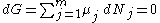For a closed system, no particles may enter or leave, although they may combine in various ways. The total number of atoms of each element will remain constant. This means that the minimization above must be subjected to the constraints: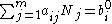where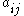is the number of atoms of element i in molecule j and bi0 is the total number of atoms of element i, which is a constant, since the system is closed. If there are a total of k types of atoms in the system, then there will be k such equations.

This is a standard problem in optimisation
Optimization (mathematics)
In mathematics, computational science, or management science, mathematical optimization refers to the selection of a best element from some set of available alternatives....

, known as constrained minimisation. The most common method of solving it is using the method of Lagrange multipliers
Lagrange multipliers
In mathematical optimization, the method of Lagrange multipliers provides a strategy for finding the maxima and minima of a function subject to constraints.For instance , consider the optimization problem...

, also known as undetermined multipliers (though other methods may be used).

Define: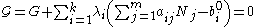where the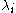are the Lagrange multipliers, one for each element. This allows each of the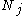to be treated independently, and it can be shown using the tools of multivariate calculus that the equilibrium condition is given by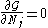and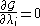(For proof see Lagrange multipliers
Lagrange multipliers
In mathematical optimization, the method of Lagrange multipliers provides a strategy for finding the maxima and minima of a function subject to constraints.For instance , consider the optimization problem...

)

This is a set of (m+k) equations in (m+k) unknowns (theand the) and may, therefore, be solved for the equilibrium concentrationsas long as the chemical potentials are known as functions of the concentrations at the given temperature and pressure. (See Thermodynamic databases for pure substances
Thermodynamic databases for pure substances
Thermodynamic databases contain information about thermodynamic properties for substances, the most important being enthalpy, entropy, and Gibbs free energy. Numerical values of these thermodynamic properties are collected as tables or are calculated from thermodynamic datafiles...

).

This method of calculating equilibrium chemical concentrations is useful for systems with a large number of different molecules. The use of k atomic element conservation equations for the mass constraint is straightforward, and replaces the use of the stoichiometric coefficient equations.

• Autocatalytic reactions and order creation
• Benesi-Hildebrand method
Benesi-Hildebrand method
The Benesi-Hildebrand method is a mathematical approach used in physical chemistry for the determination of the equilibrium constant K and stoichiometry of non-bonding interactions...

• Determination of equilibrium constants
Determination of equilibrium constants
Equilibrium constants are determined in order to quantify chemical equilibria. When an equilibrium constant is expressed as a concentration quotient,K=\frac...

• Equilibrium constant
• Henderson–Hasselbalch equation
• Isotope fractionation
Isotope fractionation
Isotope fractionation is the separation of a mixture of isotopes into its components.There are four types of isotope fractionation:* equilibrium fractionation* kinetic fractionation* transient kinetic isotope fractionation* mass-independent fractionation...

• Michaelis-Menten kinetics
Michaelis-Menten kinetics
In biochemistry, Michaelis–Menten kinetics is one of the simplest and best-known models of enzyme kinetics. It is named after German biochemist Leonor Michaelis and Canadian physician Maud Menten. The model takes the form of an equation describing the rate of enzymatic reactions, by relating...

• Redox equilibria
Standard electrode potential
In electrochemistry, the standard electrode potential, abbreviated E° or E , is the measure of individual potential of a reversible electrode at standard state, which is with solutes at an effective concentration of 1 mol dm−3, and gases at a pressure of 1 atm...

• Thermodynamic databases for pure substances
Thermodynamic databases for pure substances
Thermodynamic databases contain information about thermodynamic properties for substances, the most important being enthalpy, entropy, and Gibbs free energy. Numerical values of these thermodynamic properties are collected as tables or are calculated from thermodynamic datafiles...

• F. Van Zeggeren and S.H. Storey, The Computation of Chemical Equilibria, Cambridge University Press, 1970. Mainly concerned with gas-phase equilibria.
• D. J. Leggett (editor), Computational Methods for the Determination of Formation Constants, Plenum Press, 1985.
• A.E. Martell and R.J. Motekaitis, The Determination and Use of Stability Constants, Wiley-VCH, 1992.
• P. Gans, Stability Constants: Determination and Uses, an interactive CD, Protonic Software (Leeds), 2004

### Computer programs

There are n mass-balance equations in n unknown free concentrations. This constitutes a set of non-linear equations that must be solved by a method of successive approximations. The most commonly-used method is the Newton-Raphson method, which has been the subject of numerous publications. Some general computer programs are listed here.
• Geochem-EZ- (freeware) a multi-purpose chemical speciation program, used in plant nutrition and in soil and environmental chemistry research to perform equilibrium speciation computations, allowing the user to estimate solution ion activities and to consider simple complexes and solid phases.
• HySS Titration simulation and speciation calculations.
• EQS4WIN A powerful computer program originally developed for gas-phase equilibria but subsequently extended to general applications. Uses the Gibbs energy minimization approach.
• CHEMEQL A comprehensive computer program for the calculation of thermodynamic equilibrium concentrations of species in homogeneous and heterogeneous systems. Many geochemical applications.
• WinSGW A Windows version of the SOLGASWATER computer program.
• Visual MINTEQ A Windows version of MINTEQA2 (ver 4.0). MINTEQA2 is a chemical equilibrium model for the calculation of metal speciation, solubility equilibria etc. for natural waters.
• MINEQL+ A chemical equilibrium modeling system for aqueous systems. Handles a wide range of pH, redox, solubility and sorption scenarios.

### Software

• Aqua solution software (Sukhno Igor, Buzko Vladimir, Polushin Alexey) A set of six computer programs for
• Specific Interaction Theory. An editable database of published SIT parameters. Estimation of SIT parameters and adjustment of stability constants for changes in ionic strength.
• Calculation of electrolyte activity coefficients, ionic activity coefficients, osmotic coefficients
• Calculation of acid-base equilibria in electrolyte solutions and sea water
• Calculation of O2 solubility in water, electrolyte solutions, natural fluids, and seawater as a function of temperature, concentration, salinity, altitude, external pressure, humidity
• Prediction of temperature dependence of lg K values using various thermodynamic models
• JESS:A powerful research tool for thermodynamic and kinetic modelling of chemical speciation in complex aqueous environments, with a focus on determining thermodynamic consistency by automatic means.
• Chemical Equilibrium Calculator
• Mission to Mars – A chemistry tutorial for high school students